### Rational Numbers Class 8th Mathematics AP Board Solution

##### Question 1.Express each of the following decimal in the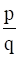form.(i) 0.57(ii) 0.176(iii) 1.00001(iv) 25.125Answer:(i)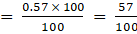(ii)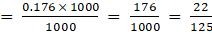(iii)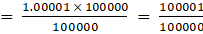(iv)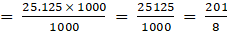Question 2.Express each of the following decimals in the rational form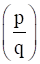.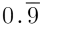Answer:let x =x = 0.99999….. -→(i)Here the periodicity of the decimal is oneSo, we multiply both sides of (i) by 10 and we get10x = 9.999…-→(ii)Subtract (i) from (ii)10x = 9.999….x = 0.999…10x - x = 9.999... - 0.999...9x = 9.0x = 1Hence= 1Question 3.Express each of the following decimals in the rational form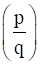.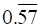Answer:let x =X = 0.575757….. -→(i)Here the periodicity of the decimal is twoSo, we multiply both sides of (i) by 100 and we get100x = 57.575757…-→(ii)Subtract (i) from (ii)100x = 57.5757….X = 0.5757…99x = 57.0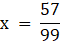(divide by 99 on both sides)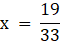Hence=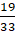Question 4.Find (x + y) ÷ (x − y) if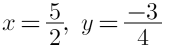Answer:According to the question we have,(x + y) ÷ (x − y)Put the values of x and y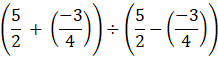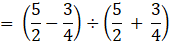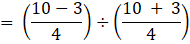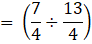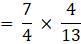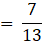Question 5.Find (x + y) ÷ (x − y) ifx =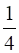, y =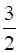Answer:According to the question we have,(x + y) ÷ (x − y)Put the values of x and y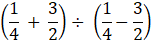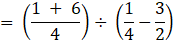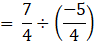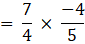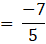Question 6.Divide the sum of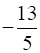and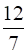by the product of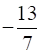and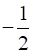.Answer:To find the sum,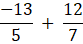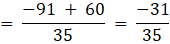To find the sum,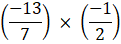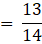According to the given question,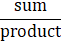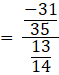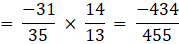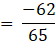Question 7.If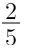of a number exceeds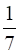of the same number by 36. Find the number.Answer:let the number is xThen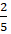of x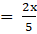According to the question,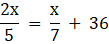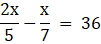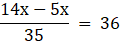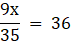9x = 36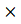35(multiply 35 on both the sides)9X = 1260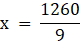(divide by 9 on both the sides)X = 140Question 8.Two pieces of lengths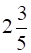m and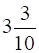m are cut off from a rope 11 m long. What is the length of the remaining rope?Answer:total length of the rope = 11mLet the third part of rope be xLength of first piece =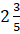m =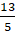mLength of second piece =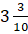m =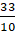mAccording to the question,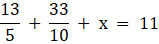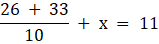(By taking L.C.M.)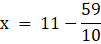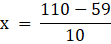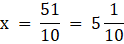Question 9.The cost of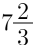meters of cloth is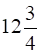. Find the cost per metre.Answer:cost of cloth =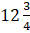=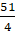Length of cloth =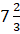=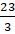Cost per meter =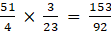(divide cost of cloth by length of cloth)= 1.66 is the cost per meterQuestion 10.Find the area of a rectangular park which is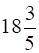m long and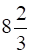m broad.Answer:length =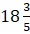m =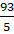Breadth =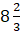m =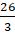Area = length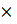breadth=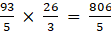Question 11.What number should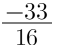be divided by to get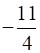?Answer:let the number which be divided by be xAccording to the question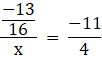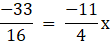(Multiply by x on both the sides)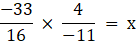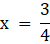Question 12.If 36 trousers of equal sizes can be stitched with 64 meters of cloth. What is the length of the cloth required for each trouser?Answer:number of trousers = 36Length of cloth = 64mLength required for each trouser =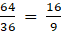mQuestion 13.When the repeating decimal 0.363636 .... is written in simplest fractional form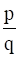, find the sum p + q.Answer:x = 0.363636…(i)Periodicity = 2So,100x = 36.363636….(ii)From (i) and (ii)100x = 36.363636….X = 0.3636363…_____________________99x = 36X =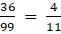Then p = 4 and q = 11p + q = 4 + 11 = 15

PDF FILE TO YOUR EMAIL IMMEDIATELY PURCHASE NOTES & PAPER SOLUTION. @ Rs. 50/- each (GST extra)

HINDI ENTIRE PAPER SOLUTION

MARATHI PAPER SOLUTION

SSC MATHS I PAPER SOLUTION

SSC MATHS II PAPER SOLUTION

SSC SCIENCE I PAPER SOLUTION

SSC SCIENCE II PAPER SOLUTION

SSC ENGLISH PAPER SOLUTION

SSC & HSC ENGLISH WRITING SKILL

HSC ACCOUNTS NOTES

HSC OCM NOTES

HSC ECONOMICS NOTES

HSC SECRETARIAL PRACTICE NOTES

# 2019 Board Paper Solution

HSC ENGLISH SET A 2019 21st February, 2019

HSC ENGLISH SET B 2019 21st February, 2019

HSC ENGLISH SET C 2019 21st February, 2019

HSC ENGLISH SET D 2019 21st February, 2019

SECRETARIAL PRACTICE (S.P) 2019 25th February, 2019

HSC XII PHYSICS 2019 25th February, 2019

CHEMISTRY XII HSC SOLUTION 27th, February, 2019

OCM PAPER SOLUTION 2019 27th, February, 2019

HSC MATHS PAPER SOLUTION COMMERCE, 2nd March, 2019

HSC MATHS PAPER SOLUTION SCIENCE 2nd, March, 2019

SSC ENGLISH STD 10 5TH MARCH, 2019.

HSC XII ACCOUNTS 2019 6th March, 2019

HSC XII BIOLOGY 2019 6TH March, 2019

HSC XII ECONOMICS 9Th March 2019

SSC Maths I March 2019 Solution 10th Standard11th, March, 2019

SSC MATHS II MARCH 2019 SOLUTION 10TH STD.13th March, 2019

SSC SCIENCE I MARCH 2019 SOLUTION 10TH STD. 15th March, 2019.

SSC SCIENCE II MARCH 2019 SOLUTION 10TH STD. 18th March, 2019.

SSC SOCIAL SCIENCE I MARCH 2019 SOLUTION20th March, 2019

SSC SOCIAL SCIENCE II MARCH 2019 SOLUTION, 22nd March, 2019

XII CBSE - BOARD - MARCH - 2019 ENGLISH - QP + SOLUTIONS, 2nd March, 2019

# HSCMaharashtraBoardPapers2020

(Std 12th English Medium)

HSC ECONOMICS MARCH 2020

HSC OCM MARCH 2020

HSC ACCOUNTS MARCH 2020

HSC S.P. MARCH 2020

HSC ENGLISH MARCH 2020

HSC HINDI MARCH 2020

HSC MARATHI MARCH 2020

HSC MATHS MARCH 2020

# SSCMaharashtraBoardPapers2020

(Std 10th English Medium)

English MARCH 2020

HindI MARCH 2020

Hindi (Composite) MARCH 2020

Marathi MARCH 2020

Mathematics (Paper 1) MARCH 2020

Mathematics (Paper 2) MARCH 2020

Sanskrit MARCH 2020

Sanskrit (Composite) MARCH 2020

Science (Paper 1) MARCH 2020

Science (Paper 2)

Geography Model Set 1 2020-2021

MUST REMEMBER THINGS on the day of Exam

Are you prepared? for English Grammar in Board Exam.

Paper Presentation In Board Exam

How to Score Good Marks in SSC Board Exams

Tips To Score More Than 90% Marks In 12th Board Exam

How to write English exams?

How to prepare for board exam when less time is left

How to memorise what you learn for board exam

No. 1 Simple Hack, you can try out, in preparing for Board Exam

How to Study for CBSE Class 10 Board Exams Subject Wise Tips?

JEE Main 2020 Registration Process – Exam Pattern & Important Dates

NEET UG 2020 Registration Process Exam Pattern & Important Dates

How can One Prepare for two Competitive Exams at the same time?

8 Proven Tips to Handle Anxiety before Exams!